## Database Functions

In this lesson you can learn about all database functions in Excel.
General Syntax:

Function_name (database, field, criteria)

• Database – range of cells, where your database is
• Field – name or numer of column where values are
• Criteria – your criteria – they should contain name of column and name of some value from that column

Let’s see train that with examples. This is example database: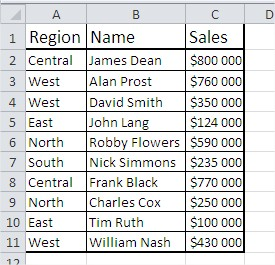# DAVERAGE

This function shows average of values which meet your criteria. This is the example:

Syntax:

=DAVERAGE(\$A\$1:\$C\$11;3;\$A\$1:\$A\$2) or =DAVERAGE(\$A\$1:\$C\$11;”Sales”;\$A\$1:\$A\$2)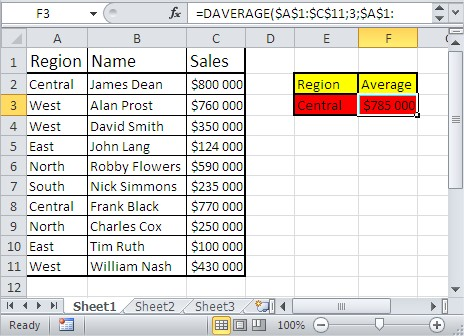# DCOUNT

This function shows count of cells which meet your criteria. This is the example:

Syntax:

=DCOUNT(\$A\$1:\$C\$11,3,\$E\$2:\$E\$3) or =DCOUNT(\$A\$1:\$C\$11,”Sales”,\$E\$2:\$E\$3)# DCOUNTA

This function shows count of noblank cells which meet your criteria. DCOUNTA function works similar to DCOUNT function. This is the example:

Syntax:

=DCOUNTA(\$A\$1:\$C\$11,3,\$E\$2:\$E\$3) or =DCOUNTA(\$A\$1:\$C\$11,”Sales”,\$E\$2:\$E\$3)# DGET

This function shows single value which meet your criteria. This is the example:

Syntax:

=DGET(\$A\$1:\$C\$11,3,A1:A2) or =DGET(\$A\$1:\$C\$11,”Sales”,A1:A2)# DMAX

This function shows max value which meet your criteria. This is the example:

Syntax:

=DMAX(\$A\$1:\$C\$11,3,\$A\$1:\$A\$2) or =DMAX(\$A\$1:\$C\$11,”Sales”,\$A\$1:\$A\$2)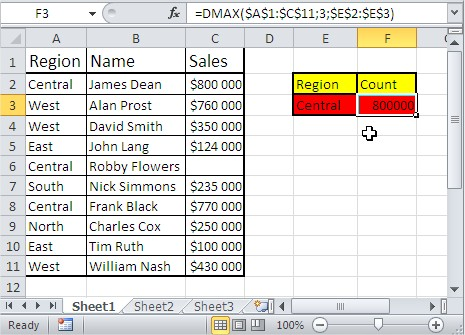# DMIN

This function shows min value which meet your criteria. This is the example:

Syntax:

=DMIN(\$A\$1:\$C\$11,3,\$A\$1:\$A\$2) or =DMIN(\$A\$1:\$C\$11,”Sales”,\$A\$1:\$A\$2)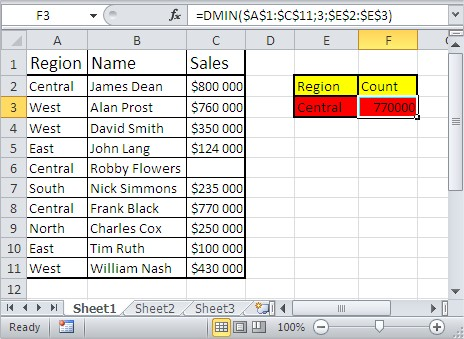# DPRODUCT

This function shows multiplication of values which meet your criteria. This is the example:

Syntax:

=DPRODUCT(\$A\$1:\$C\$11,3,\$A\$1:\$A\$2) or =DPRODUCT(\$A\$1:\$C\$11,”Sales”,\$A\$1:\$A\$2)# DSTDEV

This function estimates standard deviation of values which meet your criteria. This is the example:

Syntax:

=DSTDEV(\$A\$1:\$C\$11,3,\$A\$1:\$A\$2) or =DSTDEV(\$A\$1:\$C\$11,”Sales”,\$A\$1:\$A\$2)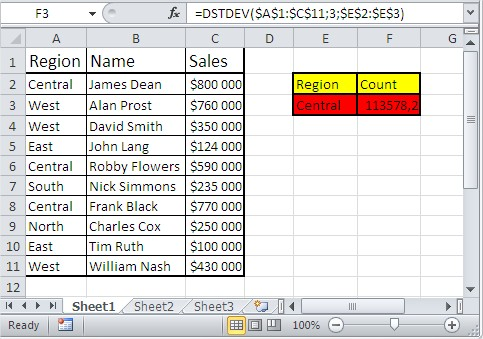# DSTDEVP

This function estimates standard deviation based of whole population of values which meet your criteria. This is the example:

Syntax:

=DSTDEVP(\$A\$1:\$C\$11,3,\$A\$1:\$A\$2) or =DSTDEVP(\$A\$1:\$C\$11,”Sales”,\$A\$1:\$A\$2)# DSUM

This function shows sum of values which meet your criteria. This is the example:

Syntax:

=DSUM(\$A\$1:\$C\$11,3,\$A\$1:\$A\$2) or =DSUM(\$A\$1:\$C\$11,”Sales”,\$A\$1:\$A\$2)# DVAR

This function estimates variance of values which meet your criteria. This is the example:

Syntax:

=DVAR(\$A\$1:\$C\$11,3,\$A\$1:\$A\$2) or =DVAR(\$A\$1:\$C\$11,”Sales”,\$A\$1:\$A\$2)# DVARP

This function estimates variance of values which meet your criteria based on entire population. This is the example:

Syntax:

=DVARP(\$A\$1:\$C\$11,3,\$A\$1:\$A\$2) or =DVARP(\$A\$1:\$C\$11,”Sales”,\$A\$1:\$A\$2)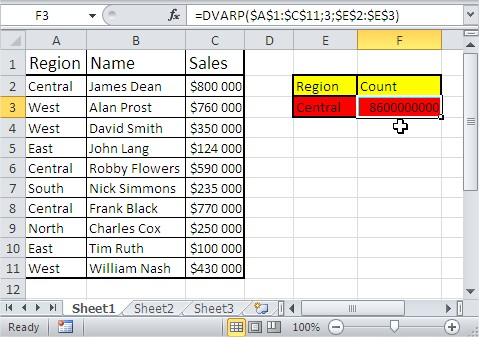## Template

```Further reading: